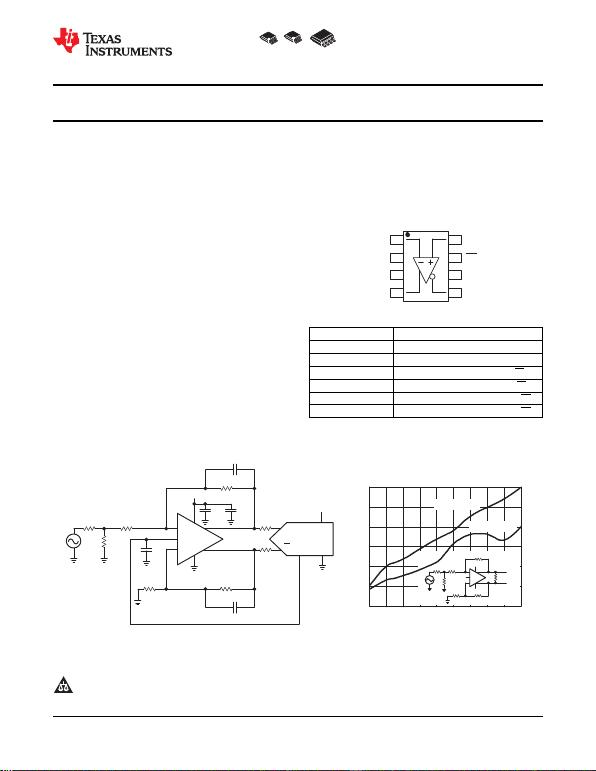DGK-8DGN-8 D-8
1
2
3
4
8
7
6
5
VIN− VIN+
VOCM
VS+
VOUT+
PD
VS−
VOUT
APPLICATION CIRCUIT DIAGRAM
f − Frequency − MHz
−80
−92
10 20 40 60
− Third-Order Intermodulation Distortion − dBc
−74
THIRD-ORDER INTERMODULATION
DISTORTION
−62
80 100
−68
−86
−98
12
10
14
16
IMD3
Bits
VS
392
+
+800
VS+ VOUT
392
402
56.2
50 374
VOCM
2.5 V
VS−
30 50 70 90
VS = 5 V
VS = ±5 V
+
+
VOCM 12 Bit/80 MSps
IN
IN
5 V
Vref
5 V
VS
0.1 µF10 µF
392
10 pF
1 µF
374
50
402
392
10 pF
24.9
24.9
THS4500
THS4501
www.ti.com
SLOS350F APRIL 2002REVISED OCTOBER 2011
WIDEBAND, LOW-DISTORTION, FULLY DIFFERENTIAL AMPLIFIERS
Check for Samples: THS4500,THS4501
1FEATURES APPLICATIONS
23Fully Differential Architecture High Linearity Analog-to-Digital Converter
Preamplifier
Bandwidth: 370 MHz Wireless Communication Receiver Chains
Slew Rate: 2800 V/μsSingle-Ended to Differential Conversion
IMD3:90 dBc at 30 MHz Differential Line Driver
OIP3: 49 dBm at 30 MHz Active Filtering of Differential Signals
Output Common-Mode Control
Wide Power-Supply Voltage Range: 5 V, ±5 V,
12 V, 15 V
Input Common-Mode Range Shifted to Include
Negative Power-Supply Rail
Power-Down Capability (THS4500)
Evaluation Module Available
DESCRIPTION RELATED DEVICES
DEVICE(1) DESCRIPTION
The THS4500 and THS4501 are high-performance
fully differential amplifiers from Texas Instruments. THS4500/1 370 MHz, 2800 V/μs, VICR Includes VS
The THS4500, featuring power-down capability, and THS4502/3 370 MHz, 2800 V/μs, Centered VICR
the THS4501, without power-down capability, set new THS4120/1 3.3 V, 100 MHz, 43 V/μs, 3.7 nV/Hz
performance standards for fully differential amplifiers THS4130/1 ±15 V, 150 MHz, 51 V/μs, 1.3 nV/Hz
with unsurpassed linearity, supporting 14-bit THS4140/1 ±15 V, 160 MHz, 450 V/μs, 6.5 nV/Hz
operation through 40 MHz. Package options include
the SOIC-8 and the MSOP-8 with PowerPADfor a THS4150/1 ±15 V, 150 MHz, 650 V/μs, 7.6 nV/Hz
smaller footprint, enhanced ac performance, and
improved thermal dissipation capability. (1) Even-numbered devices feature power-down capability.
1Please be aware that an important notice concerning availability, standard warranty, and use in critical applications of Texas
Instruments semiconductor products and disclaimers thereto appears at the end of this data sheet.
3All other trademarks are the property of their respective owners.
PRODUCTION DATA information is current as of publication date. Copyright ©20022011, Texas Instruments Incorporated
Products conform to specifications per the terms of the Texas
Instruments standard warranty. Production processing does not
necessarily include testing of all parameters.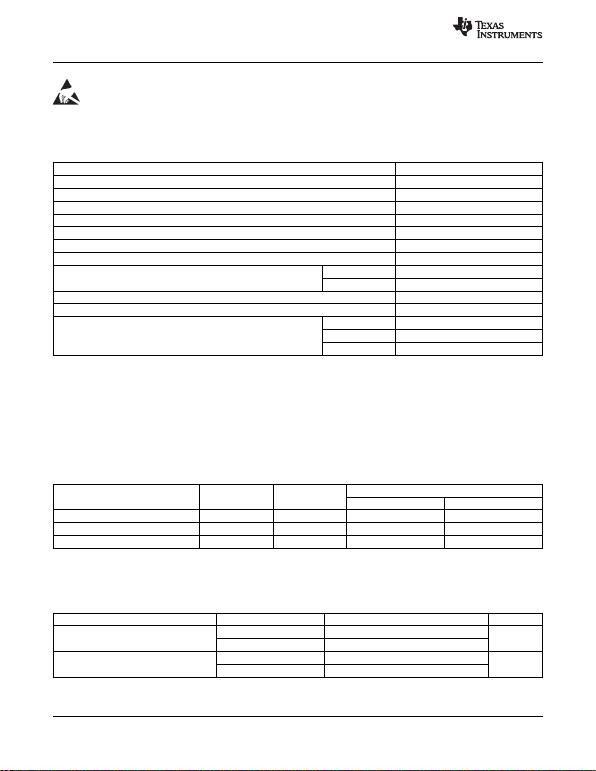THS4500
THS4501
SLOS350F APRIL 2002REVISED OCTOBER 2011
www.ti.com
This integrated circuit can be damaged by ESD. Texas Instruments recommends that all integrated circuits be handled with
appropriate precautions. Failure to observe proper handling and installation procedures can cause damage.
ESD damage can range from subtle performance degradation to complete device failure. Precision integrated circuits may be more
susceptible to damage because very small parametric changes could cause the device not to meet its published specifications.
ABSOLUTE MAXIMUM RATINGS(1)
Over operating free-air temperature range, unless otherwise noted. UNIT
Supply voltage, VS16.5 V
Input voltage, VI±VS
Output current, IO(2) 150 mA
Differential input voltage, VID 4 V
Continuous power dissipation See Dissipation Rating Table
Maximum junction temperature, TJ(3) +150°C
Maximum junction temperature, continuous operation, long-term reliability, TJ+125°C
C suffix 0°C to +70°C
Operating free-air temperature range, TA(4) I suffix 40°C to +85°C
Storage temperature range, Tstg 65°C to +150°C
Lead temperature 1,6 mm (1/16 inch) from case for 10 seconds +300°C
HBM 4000 V
ESD rating: CDM 1000 V
MM 100 V
(1) Stresses above these ratings may cause permanent damage. Exposure to absolute maximum conditions for extended periods may
degrade device reliability. These are stress ratings only, and functional operation of the device at these or any other conditions beyond
those specified is not implied.
(2) The THS4500/1 may incorporate a PowerPAD on the underside of the chip. This acts as a heat sink and must be connected to a
thermally dissipative plane for proper power dissipation. Failure to do so may result in exceeding the maximum junction temperature
which could permanently damage the device. See TI technical briefs SLMA002 and SLMA004 for more information about utilizing the
(3) The absolute maximum temperature under any condition is limited by the constraints of the silicon process.
(4) The maximum junction temperature for continuous operation is limited by package constraints. Operation above this temperature may
result in reduced reliability and/or lifetime of the device.
DISSIPATION RATINGS TABLE POWER RATING(2)
θJC θJA (1)
PACKAGE (°C/W) (°C/W) TA+25°C TA= +85°C
D (8-pin) 38.3 97.5 1.02 W 410 mW
DGN (8-pin) 4.7 58.4 1.71 W 685 mW
DGK (8-pin) 54.2 260 385 mW 154 mW
(1) This data was taken using the JEDEC standard High-K test PCB.
(2) Power rating is determined with a junction temperature of +125°C. This is the point where distortion starts to substantially increase.
Thermal management of the final PCB should strive to keep the junction temperature at or below +125°C for best performance and
long-term reliability.
RECOMMENDED OPERATING CONDITIONS MIN NOM MAX UNIT
Dual supply ±5±7.5
Supply voltage V
Single supply 4.5 5 15
C suffix 0 +70
Operating free- air temperature, TA°C
I suffix 40 +85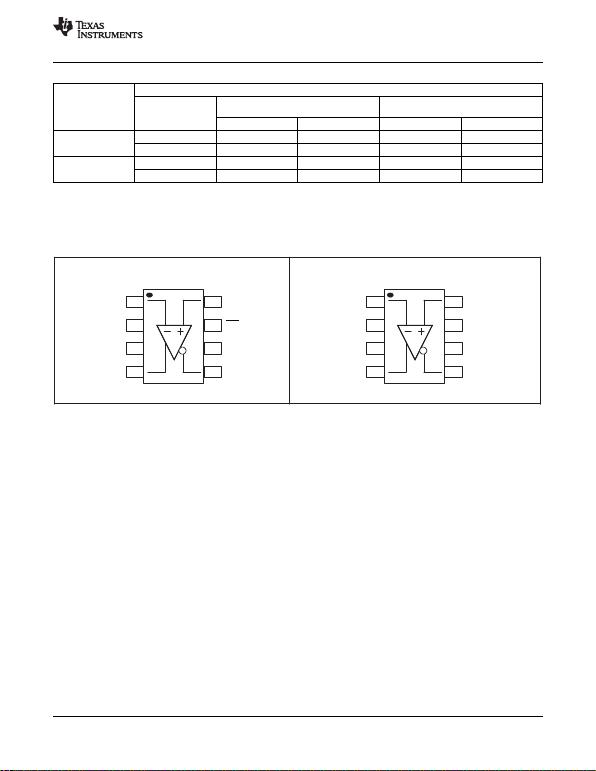(TOPVIEW)
THS4501 D,DGN,DGK
(TOPVIEW)
THS4500 D,DGN,DGK
VIN-1
2
3
4
8
7
6
5
VOCM
VS+
VOUT+
VIN+
VS-
VOUT-
PD
VIN-1
2
3
4
8
7
6
5
VOCM
VS+
VOUT+
VIN+
VS-
VOUT-
NC
THS4500
THS4501
www.ti.com
SLOS350F APRIL 2002REVISED OCTOBER 2011
PACKAGE/ORDERING INFORMATION(1)
ORDERABLE PACKAGE AND NUMBER
PLASTIC MSOP(2)
TEMPERATURE PLASTIC MSOP(2)
OUTLINE (D) DGN SYMBOL DGK SYMBOL
THS4500CD THS4500CDGN BFB THS4500CDGK ATVB
0°C to +70°CTHS4501CD THS4501CDGN BFD THS4501CDGK ATW
THS4500ID THS4500IDGN BFC THS4500IDGK ASV
40°C to +85°CTHS4501ID THS4501IDGN BFE THS4501IDGK ASW
(1) For the most current package and ordering information see the Package Option Addendum at the end of this document, or see the TI
web site at www.ti.com.
(2) All packages are available taped and reeled. The R suffix standard quantity is 2500. The T suffix standard quantity is 250 (for example,
THS4501DT).
PIN ASSIGNMENTS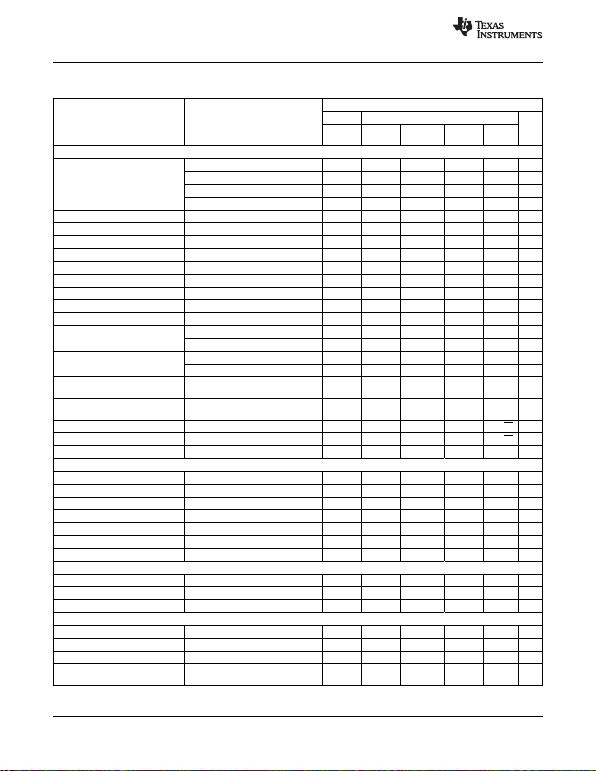THS4500
THS4501
SLOS350F APRIL 2002REVISED OCTOBER 2011
www.ti.com
ELECTRICAL CHARACTERISTICS: VS=±5 V
RF= RG= 392 , RL= 800 , G = +1, and single-ended input, unless otherwise noted.THS4500 AND THS4501
TYP OVER TEMPERATURE MIN/
PARAMETER TEST CONDITIONS TYP/
0°C to 40°C to
+25°C +25°C UNITS MAX
+70°C +85°C
AC PERFORMANCE
G = +1, PIN =20 dBm, RF= 392 370 MHz Typ
G = +2, PIN =30 dBm, RF= 1 k175 MHz Typ
Small-signal bandwidth G = +5, PIN =30 dBm, RF= 2.4 k70 MHz Typ
G = +10, PIN =30 dBm, RF= 5.1 k30 MHz Typ
Gain-bandwidth product G >+10 300 MHz Typ
Bandwidth for 0.1-dB flatness PIN =20 dBm 150 MHz Typ
Large-signal bandwidth VP= 2 V 220 MHz Typ
Slew rate 4 VPP Step 2800 V/μs Typ
Rise time 2 VPP Step 0.4 ns Typ
Fall time 2 VPP Step 0.5 ns Typ
Settling time to 0.01% VO= 4 VPP 8.3 ns Typ
0.1% VO= 4 VPP 6.3 ns Typ
Harmonic distortion G = +1, VO= 2 VPP Typ
f = 8 MHz 82 dBc Typ
2nd harmonic f = 30 MHz 71 dBc Typ
f = 8 MHz 97 dBc Typ
3rd harmonic f = 30 MHz 74 dBc Typ
Third-order intermodulation VO= 2 VPP, fC= 30 MHz, RF= 392 ,90 dBc Typ
distortion 200 kHz tone spacing
fC= 30 MHz, RF= 392 ,
Third-order output intercept point 49 dBm Typ
Referenced to 50
Input voltage noise f >1 MHz 7 nV/Hz Typ
Input current noise f >100 kHz 1.7 pA/Hz Typ
Overdrive recovery time Overdrive = 5.5 V 60 ns Typ
DC PERFORMANCE
Open-loop voltage gain 55 52 50 50 dB Min
Input offset voltage 47/18/0 9/+1 mV Max
Average offset voltage drift ±10 ±10 μV/°C Typ
Input bias current 4 4.6 5 5.2 μA Max
Average bias current drift ±10 ±10 nA/°C Typ
Input offset current 0.5 1 2 2 μA Max
Average offset current drift ±40 ±40 nA/°C Typ
INPUT
Common-mode input range 5.7/2.6 5.4/2.3 5.1/2 5.1/2 V Min
Common-mode rejection ratio 80 74 70 70 dB Min
Input impedance 107|| 1 || pF Typ
OUTPUT
Differential output voltage swing RL= 1 k ±8±7.6 ±7.4 ±7.4 V Min
Differential output current drive RL= 20 120 110 100 100 mA Min
Output balance error PIN =20 dBm, f = 100 kHz 58 dB Typ
Closed-loop output impedance f = 1 MHz 0.1 Typ
(single-ended)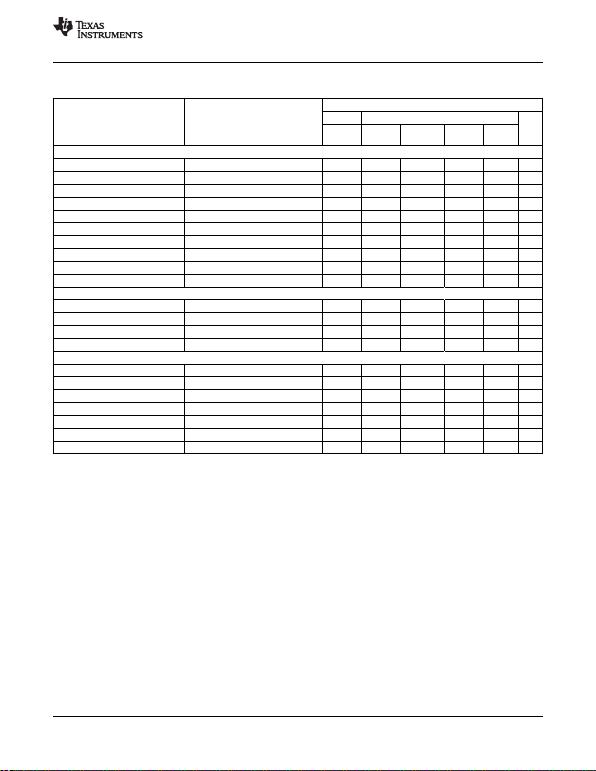THS4500
THS4501
www.ti.com
SLOS350F APRIL 2002REVISED OCTOBER 2011
ELECTRICAL CHARACTERISTICS: VS=±5 V (continued)
RF= RG= 392 , RL= 800 , G = +1, and single-ended input, unless otherwise noted.THS4500 AND THS4501
TYP OVER TEMPERATURE MIN/
PARAMETER TEST CONDITIONS TYP/
0°C to 40°C to
+25°C +25°C UNITS MAX
+70°C +85°C
OUTPUT COMMON-MODE VOLTAGE CONTROL
Small-signal bandwidth RL= 400 180 MHz Typ
Slew rate 2 VPP Step 92 V/μs Typ
Minimum gain 1 0.98 0.98 0.98 V/V Min
Maximum gain 1 1.02 1.02 1.02 V/V Max
Common-mode offset voltage 0.4 4.6/+3.8 6.6/+5.8 7.6/+6.8 mV Max
Input bias current VOCM = 2.5 V 100 150 170 170 μA Max
Input voltage range ±4±3.7 ±3.4 ±3.4 V Min
Input impedance 25 || 1 k|| pF Typ
Maximum default voltage VOCM left floating 0 0.05 0.10 0.10 V Max
Minimum default voltage VOCM left floating 0 0.05 0.10 0.10 V Min
POWER SUPPLY
Specified operating voltage ±5 7.5 7.5 7.5 V Max
Maximum quiescent current 23 28 32 34 mA Max
Minimum quiescent current 23 18 14 12 mA Min
Power-supply rejection (±PSRR) 80 76 73 70 dB Min
POWER-DOWN (THS4500 ONLY)
Enable voltage threshold Device enabled ON above 2.9 V 2.9 V Min
Disable voltage threshold Device disabled OFF below 4.3 V 4.3 V Max
Power-down quiescent current 800 1000 1200 1200 μA Max
Input bias current 200 240 260 260 μA Max
Input impedance 50 || 1 k|| pF Typ
Turn-on time delay 1000 ns Typ
Turn-off time delay 800 ns Typ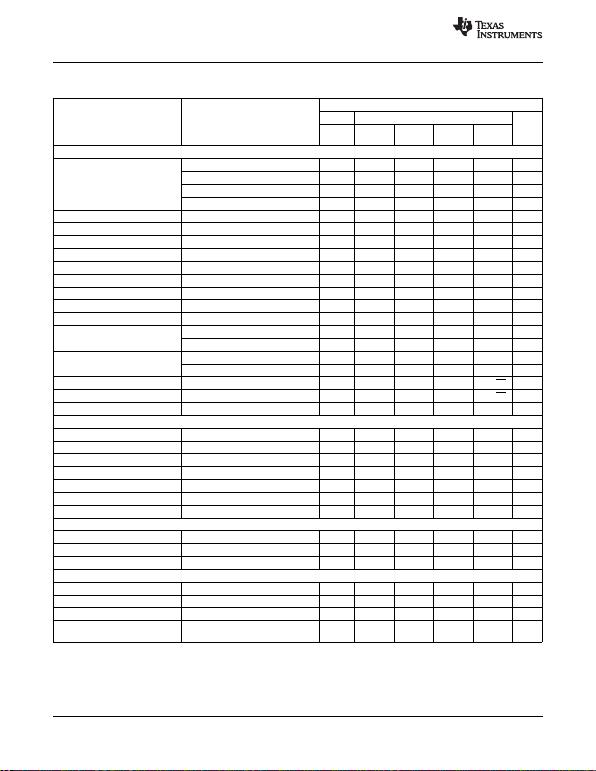THS4500
THS4501
SLOS350F APRIL 2002REVISED OCTOBER 2011
www.ti.com
ELECTRICAL CHARACTERISTICS: VS= 5 V
RF= RG= 392 , RL= 800 , G = +1, and single-ended input, unless otherwise noted.
THS4500 AND THS4501
TYP OVER TEMPERATURE MIN/T
PARAMETER TEST CONDITIONS YP/M
0°C to 40°C to
+25°C +25°C UNITS AX
+70°C +85°C
AC PERFORMANCE
G = +1, PIN =20 dBm, RF= 392 320 MHz Typ
G = +2, PIN =30 dBm, RF= 1 k160 MHz Typ
Small-signal bandwidth G = +5, PIN =30 dBm, RF= 2.4 k60 MHz Typ
G = +10, PIN =30 dBm, RF= 5.1 k30 MHz Typ
Gain-bandwidth product G >+10 300 MHz Typ
Bandwidth for 0.1-dB flatness PIN =20 dBm 180 MHz Typ
Large-signal bandwidth VP= 1 V 200 MHz Typ
Slew rate 2 VPP Step 1300 V/μs Typ
Rise time 2 VPP Step 0.5 ns Typ
Fall time 2 VPP Step 0.6 ns Typ
Settling time to 0.01% VO= 2 V Step 13.1 ns Typ
0.1% VO= 2 V Step 8.3 ns Typ
Harmonic distortion G = +1, VO= 2 VPP Typ
f = 8 MHz, 80 dBc Typ
2nd harmonic f = 30 MHz 55 dBc Typ
f = 8 MHz 76 dBc Typ
3rd harmonic f = 30 MHz 60 dBc Typ
Input voltage noise f >1 MHz 7 nV/Hz Typ
Input current noise f >100 kHz 1.7 pA/Hz Typ
Overdrive recovery time Overdrive = 5.5 V 60 ns Typ
DC PERFORMANCE
Open-loop voltage gain 54 51 49 49 dB Min
Input offset voltage 47/18/0 9/+1 mV Max
Average offset voltage drift ±10 ±10 μV/°C Typ
Input bias current 4 4.6 5 5.2 μA Max
Average bias current drift ±10 ±10 nA/°C Typ
Input offset current 0.5 0.7 1.2 1.2 μA Max
Average offset current drift ±20 ±20 nA/°C Typ
INPUT
Common-mode input range 0.7/2.6 0.4/2.3 0.1/2 0.1/2 V Min
Common-mode rejection ratio 80 74 70 70 dB Min
Input Impedance 107|| 1 || pF Typ
OUTPUT
Differential output voltage swing RL= 1 k, Referenced to 2.5 V ±3.3 ±3±2.8 ±2.8 V Min
Output current drive RL= 20 100 90 80 80 mA Min
Output balance error PIN =20 dBm, f = 100 kHz 58 dB Typ
Closed-loop output impedance f = 1 MHz 0.1 Typ
(single-ended)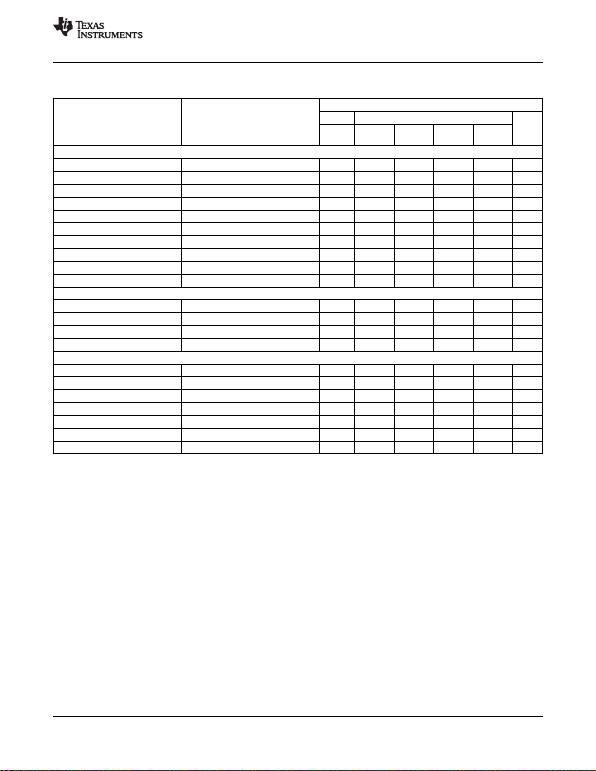THS4500
THS4501
www.ti.com
SLOS350F APRIL 2002REVISED OCTOBER 2011
ELECTRICAL CHARACTERISTICS: VS= 5 V (continued)
RF= RG= 392 , RL= 800 , G = +1, and single-ended input, unless otherwise noted.
THS4500 AND THS4501
TYP OVER TEMPERATURE MIN/T
PARAMETER TEST CONDITIONS YP/M
0°C to 40°C to
+25°C +25°C UNITS AX
+70°C +85°C
OUTPUT COMMON-MODE VOLTAGE CONTROL
Small-signal bandwidth RL= 400 180 MHz Typ
Slew rate 2 VPP Step 80 V/μs Typ
Minimum gain 1 0.98 0.98 0.98 V/V Min
Maximum gain 1 1.02 1.02 1.02 V/V Max
Common-mode offset voltage 0.4 2.6/3.4 4.2/5.4 5.6/6.4 mV Max
Input bias current VOCM = 2.5 V 1 2 3 3 μA Max
Input voltage range 1/4 1.2/3.8 1.3/3.7 1.3/3.7 V Min
Input impedance 25 || 1 k|| pF Typ
Maximum default voltage VOCM left floating 2.5 2.55 2.6 2.6 V Max
Minimum default voltage VOCM left floating 2.5 2.45 2.4 2.4 V Min
POWER SUPPLY
Specified operating voltage 5 15 15 15 V Max
Maximum quiescent current 20 25 29 31 mA Max
Minimum quiescent current 20 16 12 10 mA Min
Power-supply rejection (+PSRR) 75 72 69 66 dB Min
POWER -DOWN (THS4500 ONLY)
Enable voltage threshold Device enabled ON above 2.1 V 2.1 V Min
Disable voltage threshold Device disabled OFF below 0.7 V 0.7 V Max
Power-down quiescent current 600 800 1200 1200 μA Max
Input bias current 100 125 140 140 μA Max
Input impedance 50 || 1 k|| pF Typ
Turn-on time delay 1000 ns Typ
Turn-off time delay 800 ns Typ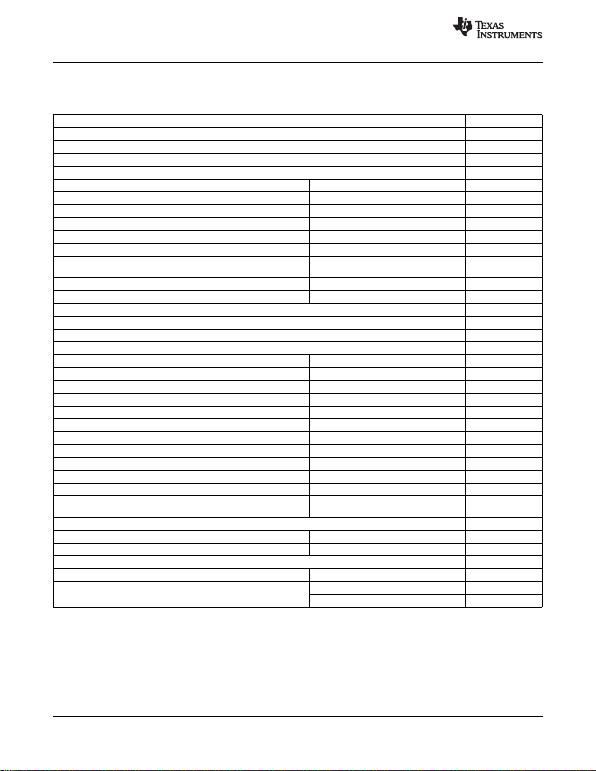THS4500
THS4501
SLOS350F APRIL 2002REVISED OCTOBER 2011
www.ti.com
TYPICAL CHARACTERISTICS
Table of Graphs (±5 V)
FIGURE
Small-signal unity-gain frequency response 1
Small-signal frequency response 2
0.1-dB gain flatness frequency response 3
Large-signal frequency response 4
Harmonic distortion (single-ended input to differential output) vs Frequency 5, 7, 13, 15
Harmonic distortion (differential input to differential output) vs Frequency 6, 8, 14, 16
Harmonic distortion (single-ended input to differential output) vs Output voltage swing 9, 11, 17, 19
Harmonic distortion (differential input to differential output) vs Output voltage swing 10, 12, 18, 20
Harmonic distortion (single-ended input to differential output) vs Load resistance 21
Harmonic distortion (differential input to differential output) vs Load resistance 22
Third-order intermodulation distortion vs Frequency 23
(single-ended input to differential output)
Third-order output intercept point vs Frequency 24
Slew rate vs Differential output voltage step 25
Settling time 26, 27
Large-signal transient response 28
Small-signal transient response 29
Overdrive recovery 30, 31
Voltage and current noise vs Frequency 32
Rejection ratios vs Frequency 33
Rejection ratios vs Case temperature 34
Output balance error vs Frequency 35
Open-loop gain and phase vs Frequency 36
Open-loop gain vs Case temperature 37
Input bias offset current vs Case temperature 38
Quiescent current vs Supply voltage 39
Input offset voltage vs Case temperature 40
Common-mode rejection ratio vs Input common-mode range 41
Output drive vs Case temperature 42
Harmonic distortion vs Output common-mode voltage 43
(single-ended and differential input to differential output)
Small-signal frequency response at VOCM 44
Output offset voltage at VOCM vs Output common-mode voltage 45
Quiescent current vs Power-down voltage 46
Turn-on and turn-off delay times 47
Single-ended output impedance in power-down vs Frequency 48
vs Case temperature 49
Power-down quiescent current vs Supply voltage 50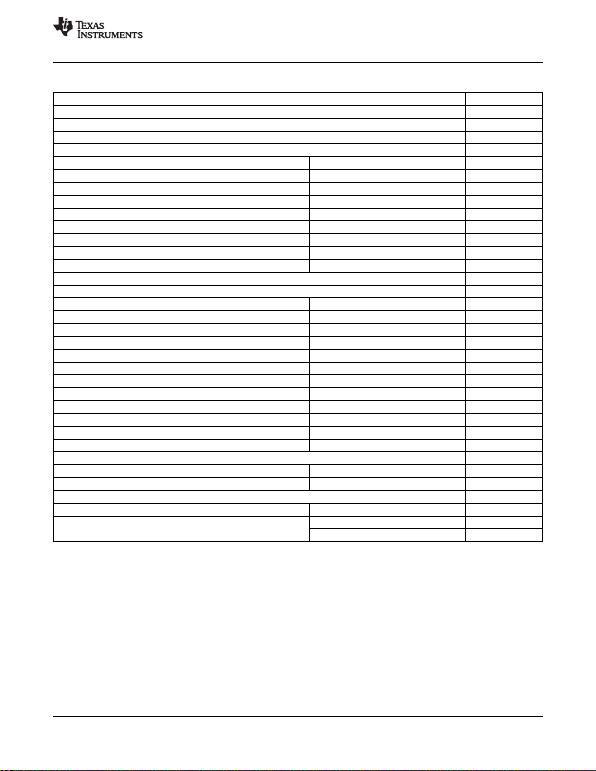THS4500
THS4501
www.ti.com
SLOS350F APRIL 2002REVISED OCTOBER 2011
Table of Graphs (5 V)
FIGURE
Small-signal unity-gain frequency response 51
Small-signal frequency response 52
0.1-dB gain flatness frequency response 53
Large-signal frequency response 54
Harmonic distortion (single-ended input to differential output) vs Frequency 55, 57, 63, 65
Harmonic distortion (differential input to differential output) vs Frequency 56, 58, 64, 66
Harmonic distortion (single-ended input to differential output) vs Output voltage swing 59, 61, 67, 69
Harmonic distortion (differential input to differential output) vs Output voltage swing 60, 62, 68, 70
Harmonic distortion (single-ended input to differential output) vs Load resistance 71
Harmonic distortion (differential input to differential output) vs Load resistance 72
Third-order intermodulation distortion vs Frequency 73
Third-order intercept point vs Frequency 74
Slew rate vs Differential output voltage step 75
Large-signal transient response 76
Small-signal transient response 77
Voltage and current noise vs Frequency 78
Rejection ratios vs Frequency 79
Rejection ratios vs Case temperature 80
Output balance error vs Frequency 81
Open-loop gain and phase vs Frequency 82
Open-loop gain vs Case temperature 83
Input bias offset current vs Case temperature 84
Quiescent current vs Supply voltage 85
Input offset voltage vs Case temperature 86
Common-mode rejection ratio vs Input common-mode range 87
Output drive vs Case temperature 88
Harmonic distortion (single-ended and differential input) vs Output common-mode voltage 89
Small-signal frequency response at VOCM 90
Output offset voltage vs Output common-mode voltage 91
Quiescent current vs Power-down voltage 92
Turn-on and turn-off delay times 93
Single-ended output impedance in power-down vs Frequency 94
vs Case temperature 95
Power-down quiescent current vs Supply voltage 96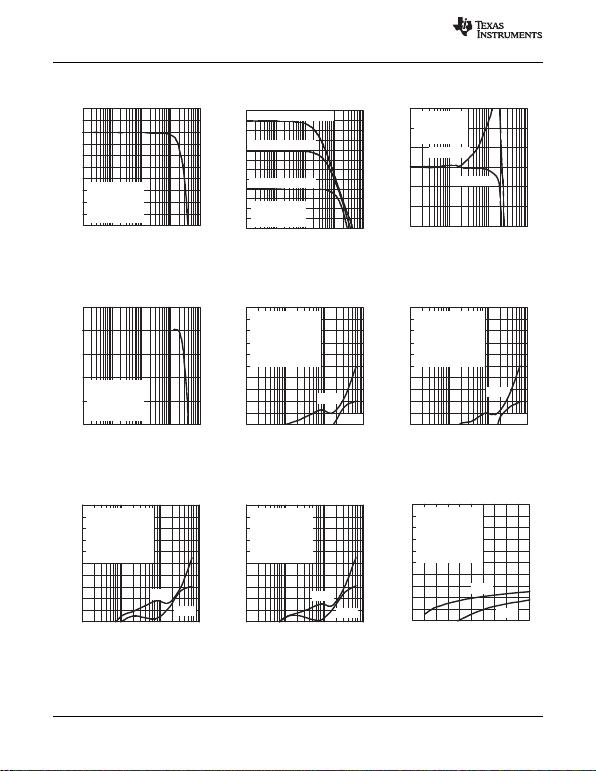−4
−3.5
−3
−2.5
−2
−1.5
−1
−0.5
0
0.5
1
0.1 1 10 100 1000
f − Frequency − MHz
Small Signal Unity Gain − dB
Gain = 1
RL = 800
Rf = 392
PIN = −20 dBm
VS = ±5 V
−0.3
−0.2
−0.1
0
0.1
0.2
0.3
110 100 1000
Rf = 392
Rf = 499
Gain = 1
RL = 800
PIN = −20 dBm
VS = ±5 V
f − Frequency − MHz
0.1 dB Gain Flatness − dB
−2
0
2
4
6
8
10
12
14
16
18
20
22
0.1 1 10 100 1000
f − Frequency − MHz
Small Signal Gain − dB
Gain = 10, Rf = 5.1 k
Gain = 5, Rf = 2.4 k
Gain = 2, Rf = 1 k
RL = 800
PIN = −30 dBm
VS = ±5 V
−100
−90
−80
−70
−60
−50
−40
−30
−20
−10
0
0.1 1 10 100
HD2
HD3
Harmonic Distortion − dBc
f − Frequency − MHz
Single-Ended Input to
Differential Output
Gain = 1
RL = 800
Rf = 392
VO = 1 VPP
VS = ±5 V
−100
−90
−80
−70
−60
−50
−40
−30
−20
−10
0
0.1 1 10 100
HD2
HD3
Harmonic Distortion − dBc
f − Frequency − MHz
Differential Input to
Differential Output
Gain = 1
RL = 800
Rf = 392
VO = 1 VPP
VS = ±5 V
−4
−3
−2
−1
0
1
0.1 1 10 100 1000
f − Frequency − MHz
Large Signal Gain − dB
Gain = 1
RL = 800
Rf = 392
PIN = 10 dBm
VS = ±5 V
−100
−90
−80
−70
−60
−50
−40
−30
−20
−10
0
0 0.5 1 1.5 2 2.5 3 3.5 4 4.5 5
HD2
HD3
Harmonic Distortion − dBc
VO − Output Voltage Swing − V
Single-Ended Input to
Differential Output
Gain = 1
RL = 800
Rf = 392
f= 8 MHz
VS = ±5 V
−100
−90
−80
−70
−60
−50
−40
−30
−20
−10
0
0.1 1 10 100
HD2
HD3
Harmonic Distortion − dBc
f − Frequency − MHz
Single-Ended Input to
Differential Output
Gain = 1
RL = 800
Rf = 392
VO = 2 VPP
VS = ±5 V
−100
−90
−80
−70
−60
−50
−40
−30
−20
−10
0
0.1 1 10 100
HD2
HD3
Harmonic Distortion − dBc
f − Frequency − MHz
Differential Input to
Differential Output
Gain = 1
RL = 800
Rf = 392
VO = 2 VPP
VS = ±5 V
THS4500
THS4501
SLOS350F APRIL 2002REVISED OCTOBER 2011
www.ti.com
TYPICAL CHARACTERISTICS: ±5 V
SMALL-SIGNAL UNITY-GAIN SMALL-SIGNAL FREQUENCY 0.1-dB GAIN FLATNESS
FREQUENCY RESPONSE RESPONSE FREQUENCY RESPONSE
Figure 1. Figure 2. Figure 3.
HARMONIC DISTORTION HARMONIC DISTORTION
LARGE-SIGNAL FREQUENCY vs vs
RESPONSE FREQUENCY FREQUENCY
Figure 4. Figure 5. Figure 6.
HARMONIC DISTORTION HARMONIC DISTORTION HARMONIC DISTORTION
vs vs vs
FREQUENCY FREQUENCY OUTPUT VOLTAGE SWING
Figure 7. Figure 8. Figure 9.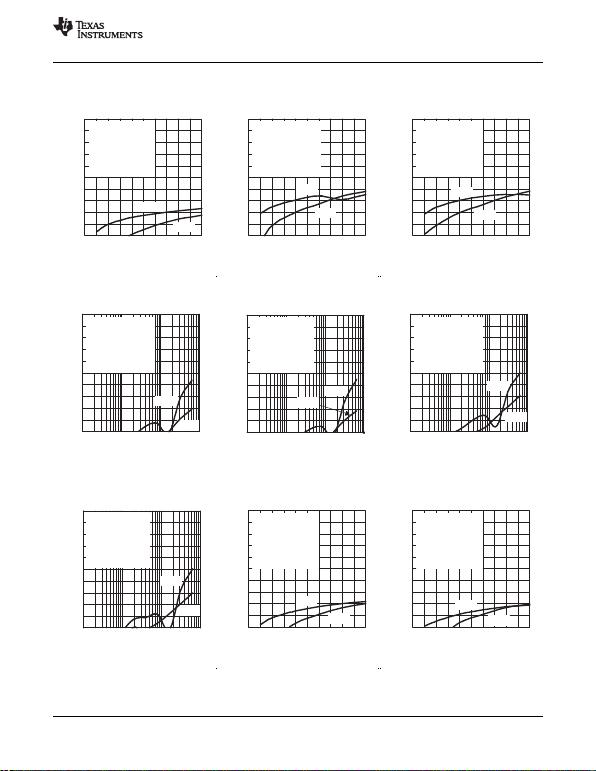−100
−90
−80
−70
−60
−50
−40
−30
−20
−10
0
0 0.5 1 1.5 2 2.5 3 3.5 4 4.5 5
HD2
HD3
Harmonic Distortion − dBc
VO − Output Voltage Swing − V
Differential Input to
Differential Output
Gain = 1
RL = 800
Rf = 499
f= 8 MHz
VS = ±5 V
−100
−90
−80
−70
−60
−50
−40
−30
−20
−10
0
0 0.5 1 1.5 2 2.5 3 3.5 4 4.5 5
HD2
HD3
Harmonic Distortion − dBc
VO − Output Voltage Swing − V
Single-Ended Input to
Differential Output
Gain = 1
RL = 800
Rf = 392
f= 30 MHz
VS = ±5 V
−100
−90
−80
−70
−60
−50
−40
−30
−20
−10
0
0 0.5 1 1.5 2 2.5 3 3.5 4 4.5 5
HD2
HD3
Harmonic Distortion − dBc
VO − Output Voltage Swing − V
Differentia Input to
Differential Output
Gain = 1
RL = 800
Rf = 392
f= 30 MHz
VS = ±5 V
−100
−90
−80
−70
−60
−50
−40
−30
−20
−10
0
0.1 1 10 100
HD2
HD3
Harmonic Distortion − dBc
f − Frequency − MHz
Single-Ended Input to
Differential Output
Gain = 2
RL = 800
Rf = 1 k
VO = 1 VPP
VS = ±5 V
−100
−90
−80
−70
−60
−50
−40
−30
−20
−10
0.1 1 10 100
0
HD2
Harmonic Distortion − dBc
f − Frequency − MHz
Differential Input to
Differential Output
Gain = 2
RL = 800
Rf = 1 k
VO = 1 VPP
VS = ±5 V
HD3
−100
−90
−80
−70
−60
−50
−40
−30
−20
−10
0.1 1 10 100
0
HD2
HD3
Harmonic Distortion − dBc
f − Frequency − MHz
Differential Input to
Differential Output
Gain = 2
RL = 800
Rf = 1 k
VO = 2 VPP
VS = ±5 V
−100
−90
−80
−70
−60
−50
−40
−30
−20
−10
0
0 0.5 1 1.5 2 2.5 3 3.5 4 4.5 5
HD2
HD3
Harmonic Distortion − dBc
VO − Output Voltage Swing − V
Single-Ended Input to
Differential Output
Gain = 2
RL = 800
Rf = 1 k
f= 8 MHz
VS = ±5 V
−100
−90
−80
−70
−60
−50
−40
−30
−20
−10
0
0 0.5 1 1.5 2 2.5 3 3.5 4 4.5 5
HD2
HD3
Harmonic Distortion − dBc
VO − Output Voltage Swing − V
Single-Ended Input to
Differential Output
Gain = 2
RL = 800
Rf = 1 k
f= 8 MHz
VS = ±5 V
THS4500
THS4501
www.ti.com
SLOS350F APRIL 2002REVISED OCTOBER 2011
TYPICAL CHARACTERISTICS: ±5 V (continued)
HARMONIC DISTORTION HARMONIC DISTORTION HARMONIC DISTORTION
vs vs vs
OUTPUT VOLTAGE SWING OUTPUT VOLTAGE SWING OUTPUT VOLTAGE SWING
Figure 10. Figure 11. Figure 12.
HARMONIC DISTORTION HARMONIC DISTORTION HARMONIC DISTORTION
vs vs vs
FREQUENCY FREQUENCY FREQUENCY
Figure 13. Figure 14. Figure 15.
HARMONIC DISTORTION HARMONIC DISTORTION HARMONIC DISTORTION
vs vs vs
FREQUENCY OUTPUT VOLTAGE SWING OUTPUT VOLTAGE SWING
Figure 16. Figure 17. Figure 18.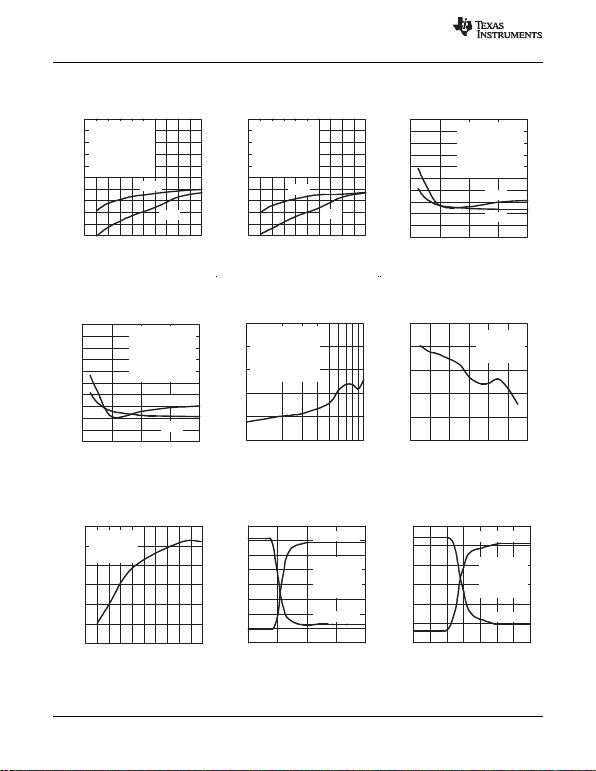−100
−90
−80
−70
−60
−50
−40
−30
−20
−10
0
0 0.5 1 1.5 2 2.5 3 3.5 4 4.5 5
HD2
HD3
Harmonic Distortion − dBc
VO − Output Voltage Swing − V
Single-Ended Input to
Differential Output
Gain = 2
RL = 800
Rf = 1 k
f= 30 MHz
VS = ±5 V
−100
−90
−80
−70
−60
−50
−40
−30
−20
−10
0
0 0.5 1 1.5 2 2.5 3 3.5 4 4.5 5
HD2
HD3
Harmonic Distortion − dBc
VO − Output Voltage Swing − V
Differentia Input to
Differential Output
Gain = 2
RL = 800
Rf = 1 k
f= 8 MHz
VS = ±5 V
−100
−90
−80
−70
−60
−50
−40
−30
−20
−10
0
0 400 800 1200 1600
HD2
HD3
Harmonic Distortion − dBc
Single-Ended Input to
Differential Output
Gain = 1
VO = 2 VPP
Rf = 392
f= 30 MHz
VS = ±5 V
30
35
40
45
50
55
0 20 40 60 80 100 120
Third-OrderOutputInterceptPoint dBm-
f Frequency MHz- -
Gain = 1
RF= 392 W
VO= 2 VPP
VS=±5 V
−100
−90
−80
−70
−60
−50
−40
−30
−20
−10
0
0 400 800 1200 1600
HD2
HD3
Harmonic Distortion − dBc
Differential Input to
Differential Output
Gain = 1
VO = 2 VPP
Rf = 392
f= 30 MHz
VS = ±5 V
−100
−90
−80
−70
−60
−50
10 100
Third-Order Intermodulation Distortion − dBc
f − Frequency − MHz
Single-Ended Input to
Differential Output
Gain = 1
RL = 800
Rf = 392
VO = 2 VPP
VS = ±5 V
-1.5
-1.0
-0.5
0
0.5
1.0
1.5
0 2 4 6 8 10 12 14
t Time ns- -
VOOutputVoltage V- -
Gain = 1
RL= 800 W
RF= 499 W
f= 1MHz
VS=±5 V
Rising Edge
Falling Edge
0 5 10 15 20
t − Time − ns
− Output Voltage − VVO
Gain = 1
RL = 800
Rf = 499
f= 1 MHz
VS = ±5 V
Rising Edge
Falling Edge
−0.8
−0.6
−0.4
−0.2
0
0.2
0.4
0.6
0.8
0
500
1000
1500
2000
2500
3000
VO − Differential Output Voltage Step − V
SR − Slew Rate − sµ
V/
Gain = 1
RL = 800
Rf = 392
VS = ±5 V
0.5 1 1.5 2 2.5 3 3.5 4 4.5 50
THS4500
THS4501
SLOS350F APRIL 2002REVISED OCTOBER 2011
www.ti.com
TYPICAL CHARACTERISTICS: ±5 V (continued)
HARMONIC DISTORTION HARMONIC DISTORTION HARMONIC DISTORTION
vs vs vs
OUTPUT VOLTAGE SWING OUTPUT VOLTAGE SWING LOAD RESISTANCE
Figure 19. Figure 20. Figure 21.
THIRD-ORDER INTERMODULATION THIRD-ORDER OUTPUT INTERCEPT
HARMONIC DISTORTION DISTORTION POINT
vs vs vs